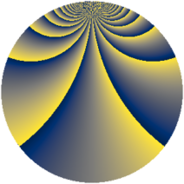# Properties

 Label 1152.3.bnLevel $1152$ Weight $3$ Character orbit 1152.bn Rep. character $\chi_{1152}(53,\cdot)$ Character field $\Q(\zeta_{32})$ Dimension $2048$ Sturm bound $576$

# Learn more

## Defining parameters

 Level: $$N$$ $$=$$ $$1152 = 2^{7} \cdot 3^{2}$$ Weight: $$k$$ $$=$$ $$3$$ Character orbit: $$[\chi]$$ $$=$$ 1152.bn (of order $$32$$ and degree $$16$$) Character conductor: $$\operatorname{cond}(\chi)$$ $$=$$ $$384$$ Character field: $$\Q(\zeta_{32})$$ Sturm bound: $$576$$

## Dimensions

The following table gives the dimensions of various subspaces of $$M_{3}(1152, [\chi])$$.

Total New Old
Modular forms 6208 2048 4160
Cusp forms 6080 2048 4032
Eisenstein series 128 0 128

## Trace form

 $$2048q + O(q^{10})$$ $$2048q - 2112q^{52} + 384q^{64} + 2688q^{70} + 832q^{76} + O(q^{100})$$

## Decomposition of $$S_{3}^{\mathrm{new}}(1152, [\chi])$$ into newform subspaces

The newforms in this space have not yet been added to the LMFDB.

## Decomposition of $$S_{3}^{\mathrm{old}}(1152, [\chi])$$ into lower level spaces

$$S_{3}^{\mathrm{old}}(1152, [\chi]) \cong$$ $$S_{3}^{\mathrm{new}}(384, [\chi])$$$$^{\oplus 2}$$